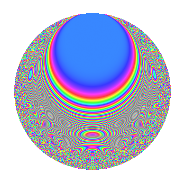# Properties

 Label 8041.2.a.jLevel 8041 Weight 2 Character orbit 8041.a Self dual Yes Analytic conductor 64.208 Analytic rank 0 Dimension 82 CM No

# Related objects

## Newspace parameters

 Level: $$N$$ = $$8041 = 11 \cdot 17 \cdot 43$$ Weight: $$k$$ = $$2$$ Character orbit: $$[\chi]$$ = 8041.a (trivial)

## Newform invariants

 Self dual: Yes Analytic conductor: $$64.2077082653$$ Analytic rank: $$0$$ Dimension: $$82$$ Fricke sign: $$-1$$ Sato-Tate group: $\mathrm{SU}(2)$

## $q$-expansion

The dimension is sufficiently large that we do not compute an algebraic $$q$$-expansion, but we have computed the trace expansion.

 $$\operatorname{Tr}(f)(q) =$$ $$82q + 8q^{2} + 6q^{3} + 98q^{4} + 11q^{5} + 10q^{6} + 8q^{7} + 30q^{8} + 108q^{9} + O(q^{10})$$ $$\operatorname{Tr}(f)(q) =$$ $$82q + 8q^{2} + 6q^{3} + 98q^{4} + 11q^{5} + 10q^{6} + 8q^{7} + 30q^{8} + 108q^{9} + q^{10} + 82q^{11} + 3q^{12} + 26q^{13} + 17q^{14} + 66q^{15} + 122q^{16} + 82q^{17} + 18q^{18} + 12q^{19} + 9q^{20} + 22q^{21} + 8q^{22} + 50q^{23} + 15q^{24} + 117q^{25} + 36q^{26} + 30q^{27} + 11q^{28} + 33q^{29} - 26q^{30} + 40q^{31} + 58q^{32} + 6q^{33} + 8q^{34} + 16q^{35} + 160q^{36} + 31q^{37} + 18q^{38} + 41q^{39} - 29q^{40} + 42q^{41} - 51q^{42} + 82q^{43} + 98q^{44} - 2q^{45} - 19q^{46} + 84q^{47} - 46q^{48} + 136q^{49} + 59q^{50} + 6q^{51} + 45q^{52} + 83q^{53} + 24q^{54} + 11q^{55} + 21q^{56} + 23q^{57} + 14q^{58} + 96q^{59} + 184q^{60} - 6q^{61} - 23q^{62} + 8q^{63} + 148q^{64} + 5q^{65} + 10q^{66} + 78q^{67} + 98q^{68} + 61q^{69} - 3q^{70} + 155q^{71} + 50q^{72} - 23q^{73} + 10q^{74} - 19q^{75} + 44q^{76} + 8q^{77} - 27q^{78} + 31q^{79} + 19q^{80} + 150q^{81} - 12q^{82} + 54q^{83} + 8q^{84} + 11q^{85} + 8q^{86} + 20q^{87} + 30q^{88} + 25q^{89} - 81q^{90} - 14q^{91} + 60q^{92} + 36q^{93} + 19q^{94} + 111q^{95} - 6q^{96} + 2q^{97} - 5q^{98} + 108q^{99} + O(q^{100})$$

## Embeddings

For each embedding $$\iota_m$$ of the coefficient field, the values $$\iota_m(a_n)$$ are shown below.

For more information on an embedded modular form you can click on its label.

Label $$a_{2}$$ $$a_{3}$$ $$a_{4}$$ $$a_{5}$$ $$a_{6}$$ $$a_{7}$$ $$a_{8}$$ $$a_{9}$$ $$a_{10}$$
1.1 −2.77655 −0.377305 5.70922 −1.07900 1.04760 −3.82470 −10.2988 −2.85764 2.99589
1.2 −2.70525 −2.86444 5.31840 1.46004 7.74904 −3.90191 −8.97712 5.20501 −3.94978
1.3 −2.65190 1.08303 5.03256 0.484312 −2.87208 1.56821 −8.04203 −1.82705 −1.28435
1.4 −2.62978 1.21378 4.91575 1.03630 −3.19199 4.97586 −7.66780 −1.52673 −2.72523
1.5 −2.57949 2.85867 4.65376 3.17304 −7.37390 −3.48304 −6.84534 5.17198 −8.18483
1.6 −2.55417 −3.23448 4.52378 −3.35627 8.26140 1.37818 −6.44616 7.46183 8.57249
1.7 −2.40536 −1.47389 3.78577 −0.987275 3.54525 2.06145 −4.29541 −0.827635 2.37475
1.8 −2.34655 3.28622 3.50630 3.73933 −7.71129 3.13477 −3.53461 7.79927 −8.77452
1.9 −2.30854 −0.184135 3.32934 −0.542742 0.425082 −0.304747 −3.06883 −2.96609 1.25294
1.10 −2.30649 −1.79768 3.31992 3.97246 4.14634 2.36407 −3.04439 0.231661 −9.16247
1.11 −2.26888 0.654424 3.14780 −0.968068 −1.48481 1.81018 −2.60421 −2.57173 2.19643
1.12 −2.25270 2.53862 3.07467 −2.69802 −5.71876 −2.07248 −2.42091 3.44461 6.07784
1.13 −2.22059 −3.23881 2.93104 −1.33112 7.19209 −0.932335 −2.06746 7.48990 2.95587
1.14 −2.00119 −0.510214 2.00475 2.33538 1.02103 −3.93653 −0.00949577 −2.73968 −4.67353
1.15 −1.99568 2.01372 1.98276 2.98897 −4.01875 −3.65858 0.0344145 1.05506 −5.96504
1.16 −1.88314 −1.39410 1.54622 −3.71860 2.62529 0.268017 0.854526 −1.05649 7.00266
1.17 −1.73828 2.71256 1.02162 −1.00305 −4.71518 1.91856 1.70071 4.35796 1.74357
1.18 −1.70166 2.90991 0.895633 −0.762355 −4.95167 4.85107 1.87925 5.46758 1.29727
1.19 −1.64446 −1.94286 0.704260 −2.95674 3.19496 4.77511 2.13080 0.774694 4.86225
1.20 −1.62861 −2.08850 0.652359 −3.32951 3.40134 −5.07571 2.19478 1.36181 5.42246
See all 82 embeddings
 $$n$$: e.g. 2-40 or 990-1000 Embeddings: e.g. 1-3 or 1.82 Significant digits: Format: Complex embeddings Normalized embeddings Satake parameters Satake angles

## Inner twists

This newform does not have CM; other inner twists have not been computed.

## Atkin-Lehner signs

$$p$$ Sign
$$11$$ $$-1$$
$$17$$ $$-1$$
$$43$$ $$-1$$

## Hecke kernels

This newform can be constructed as the kernel of the linear operator $$T_{2}^{82} - \cdots$$ acting on $$S_{2}^{\mathrm{new}}(\Gamma_0(8041))$$.